# 社交网络分析的 R 基础：（五）图的导入与简单分析

## 图的文件表示

1	2	1
1	3	-1
2	3	-1
1	4	-1
3	4	1


💡 提示

1. Stanford Large Network Dataset Collection：http://snap.stanford.edu/data

2. The KONECT Project：http://konect.cc/networks

## 导入一个图

% sym unweighted
9	4
10	6
10	7
11	1
......


graph.edges <- read.table(file = "out.dolphins", header = FALSE)


💡 提示

> class(graph.edges)
 "data.frame"


data.frame 似乎前面的章节并没有介绍，受限于研究的方向，这有可能是你唯一一次接触数据框类型，不用管它，下面将读入的数据转换为图：

> library(igraph)
> graph <- graph_from_data_frame(graph.edges, directed = FALSE)


> class(graph)
 "igraph"
> plot(graph)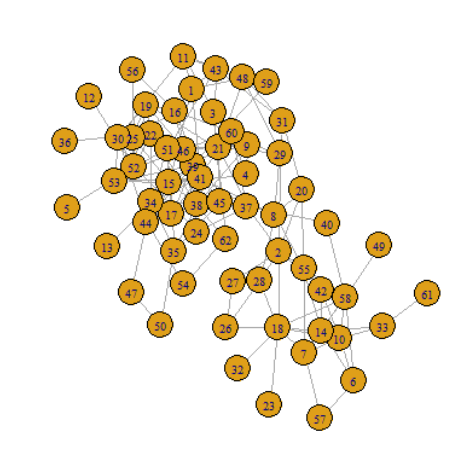> cat(sprintf("Nodes: %s\nEdges: %s\n", length(V(graph)), length(E(graph))))
Nodes: 62
Edges: 159


> save(graph, file = "dolphins.RData")  # 保存 graph 变量
> load(file = "dolphins.RData")  # 导入 RData 文件中存储的变量


## 生成人工网络

1. 全连接图
graph <- make_full_graph(10)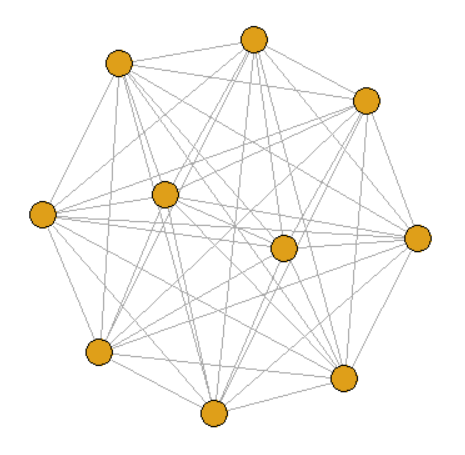2. 树状图
graph <- make_tree(21, children = 3, mode = "undirected")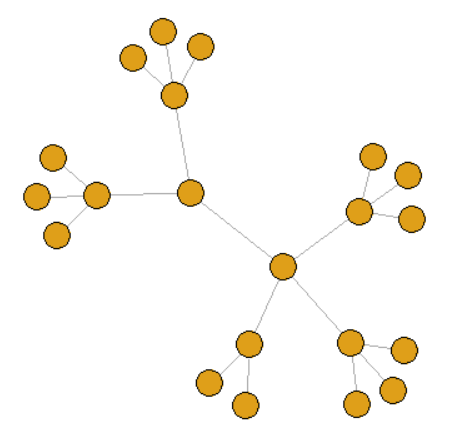3. k-正则图
graph <- sample_k_regular(20, 3)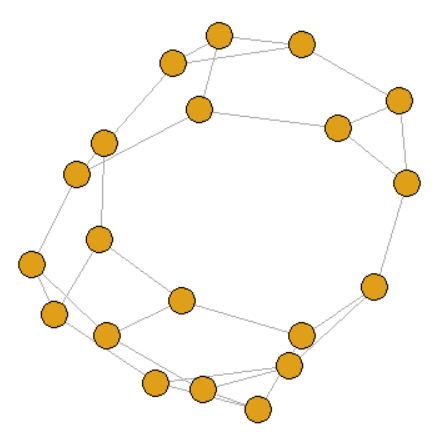4. Erdos-Renyi Random
graph <- sample_gnp(20, 0.1)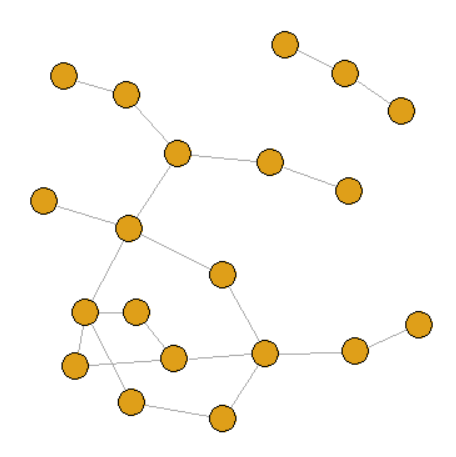5. 小世界网络
graph <- sample_smallworld(dim = 1, size = 20, nei = 2, p = 0.1)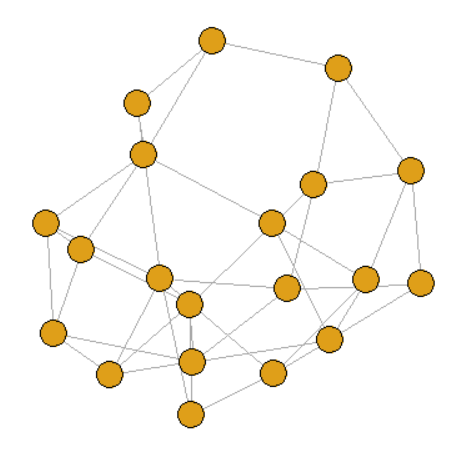💡 提示

## 图的基本分析

1. 判断图的连通性
> is.connected(graph)
 TRUE

2. 计算图的度
> degree(graph)  # 计算图中所有节点的度，其中第一行为节点的名称，第二行为节点的度
9 10 11  ...
6  7  5  ...
> degree(graph, v = "9")  # 计算图中部分节点的度
9
6

3. 计算图的密度
> edge_density(graph)
 0.0840825

4. 对图的路径分析
> diameter(graph, directed = FALSE, weights = NA)  # 直径
 8
 5
> distances(graph, v = V(graph)$name, to = V(graph)$name, algorithm = "unweighted", weights = NA)  # 计算节点间的最短距离
20
9  3
> shortest_paths(graph, from = "1", to = "6", weights = NA)  # 计算节点1到节点6的最短路径
$vpath$vpath[]
+ 6/62 vertices, named, from e1ce364:
 1  41 37 40 58 6

5. 计算图的聚类系数
> transitivity(graph, type = "average")
 0.3029323


✏️ 练习

1. 试着在数据集网站中下载其他网络导入到 R 程序中；

2. 试着计算导入网络的平均度；

3. 查找 igraph 文档，试着计算导入网络的同配系数（Assortativity）。

posted @ 2022-02-11 21:58  张高兴  阅读(378)  评论(0编辑  收藏  举报